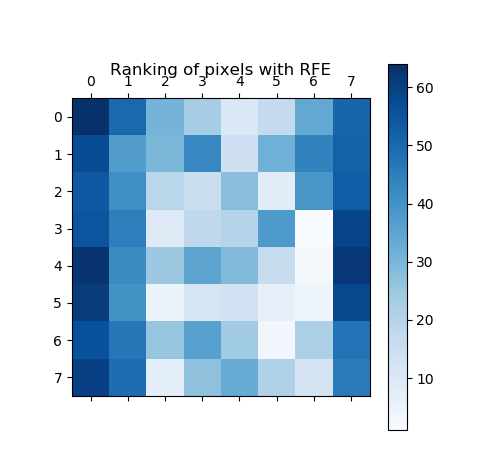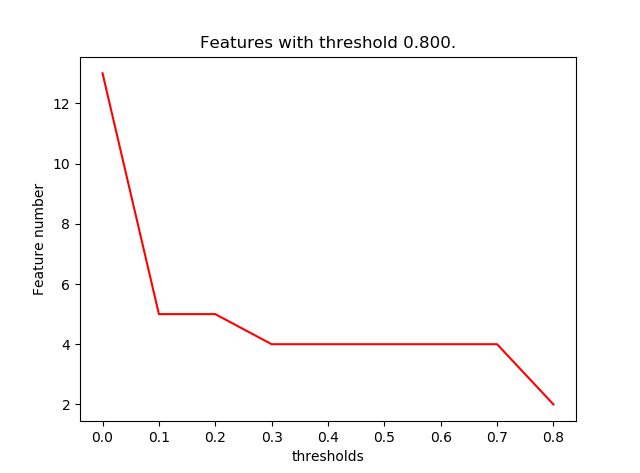# python机器学习库sklearn——特征选择

python数据挖掘系列教程

## 移除低方差特征

VarianceThreshold 是特征选择的一个简单基本方法，它会移除所有那些方差不满足一些阈值的特征。默认情况下，它将会移除所有的零方差特征，即那些在所有的样本上的取值均不变的特征。

# ============去除方差小于阈值的特征============
from sklearn.feature_selection import VarianceThreshold  #移除低方差特征
from sklearn.datasets import load_iris  # 引入花卉数据集
iris = load_iris()
X= iris.data
print(X.shape)
print(X.var(axis=0))

sel = VarianceThreshold(threshold=0.2)
X_transformed=sel.fit_transform(X)
print('去除低方差特征：\n',X_transformed.shape)


## 单变量特征选择

• SelectKBest 移除那些除了评分最高的 K 个特征之外的所有特征
• SelectPercentile 移除除了用户指定的最高得分百分比之外的所有特征
• 对每个特征应用常见的单变量统计测试: 假阳性率（false positive rate） SelectFpr, 伪发现率（false discovery rate） SelectFdr , 或者族系误差（family wise error） SelectFwe 。
• GenericUnivariateSelect 允许使用可配置方法来进行单变量特征选择。它允许超参数搜索评估器来选择最好的单变量特征。
# ============排序选择优秀特征============
from sklearn.datasets import load_iris
from sklearn.feature_selection import SelectKBest
from sklearn.feature_selection import chi2  # 引入卡方检验统计量
# 对于回归: f_regression , mutual_info_regression
# 对于分类: chi2 , f_classif , mutual_info_classif
iris = load_iris()
X, y = iris.data, iris.target
print('源样本维度：',X.shape)

X_new = SelectKBest(chi2, k=2).fit_transform(X, y)
print('新样本维度：',X_new.shape)


## 递归式特征消除

# ============递归式特征消除============
# 这里递归的移除最不重要的像素点来对每个像素点（特征）进行排序
from sklearn.svm import SVC
from sklearn.datasets import load_digits
from sklearn.feature_selection import RFE
import matplotlib.pyplot as plt

digits = load_digits()  # 加载手写体数据集
X = digits.images.reshape((len(digits.images), -1))
y = digits.target

# 创建ref对象和每个像素点的重要度排名
svc = SVC(kernel="linear", C=1)
rfe = RFE(estimator=svc, n_features_to_select=1, step=1)
rfe.fit(X, y)
ranking = rfe.ranking_.reshape(digits.images.shape)

# 绘制像素点排名
plt.matshow(ranking, cmap=plt.cm.Blues)
plt.colorbar()
plt.title("Ranking of pixels with RFE")
plt.show()## 使用 SelectFromModel 选取特征

SelectFromModel 是一个 meta-transformer（元转换器） ，它可以用来处理任何带有 coef_ 或者 feature_importances_ 属性的训练之后的评估器。 如果相关的coef_ 或者 featureimportances 属性值低于预先设置的阈值，这些特征将会被认为不重要并且移除掉。除了指定数值上的阈值之外，还可以通过给定字符串参数来使用内置的启发式方法找到一个合适的阈值。可以使用的启发式方法有 mean 、 median 以及使用浮点数乘以这些（例如，0.1*mean ）。

# ============使用 SelectFromModel 选取特征============

import matplotlib.pyplot as plt
from sklearn.datasets import load_boston
from sklearn.feature_selection import SelectFromModel
from sklearn.linear_model import LassoCV
boston = load_boston()  # 加载波士顿房价回归数据
X, y = boston['data'], boston['target']  # 取特征数据和输出数据
n_features =  # 记录循环中的特征个数，最开始数据集是有13个特征的
thresholds=  # 记录门限值，最开始是没有门限值的

clf = LassoCV()  # 使用Lasso回归

# 设置最小门限为0.25。coef_ 或者 featureimportances 属性值低于门限的都会被去除调
sfm = SelectFromModel(clf, threshold=0.1)
sfm.fit(X, y)  # 训练模型。找出模型回归系数。
X_transform = sfm.transform(X) # 根据回归系数、门限，变换数据集。
n_feature =X_transform.shape  # 获取训练以后的特征数目
n_features.append(n_feature)
thresholds.append(0.1)
while n_feature > 2:  # 如果特征数大于2，则从新转换，找最好的两个特征
sfm.threshold += 0.1  # 逐渐增加门限，进一步减少特征数目
X_transform = sfm.transform(X) # 变换数据集
n_feature = X_transform.shape
n_features.append(n_feature)  # 记录训练以后的特征数目
thresholds.append(sfm.threshold)  # 记录门限值

plt.title("Features with threshold %0.3f." % sfm.threshold)
plt.plot(thresholds, n_features, 'r')
plt.xlabel("thresholds")
plt.ylabel("Feature number")
plt.show()## 基于 L1 的特征选取

Linear models 使用 L1 正则化的线性模型会得到稀疏解：他们的许多系数为 0。 当目标是降低使用另一个分类器的数据集的维度， 它们可以与 feature_selection.SelectFromModel 一起使用来选择非零系数。特别的，可以用于此目的的稀疏评估器有用于回归的 linear_model.Lasso , 以及用于分类的 linear_model.LogisticRegression 和 svm.LinearSVC。

# ============基于 L1 的特征选取============
from sklearn.svm import LinearSVC
from sklearn.datasets import load_iris
from sklearn.feature_selection import SelectFromModel
iris = load_iris()
X, y = iris.data, iris.target
print('原数据集维度：',X.shape)
lsvc = LinearSVC(C=0.01, penalty="l1", dual=False).fit(X, y)
model = SelectFromModel(lsvc, prefit=True)
X_new = model.transform(X)
print('新数据集维度：',X_new.shape)

## 基于 Tree（树）的特征选取

# ============基于 Tree（树）的特征选取============
from sklearn.ensemble import ExtraTreesClassifier
from sklearn.datasets import load_iris
from sklearn.feature_selection import SelectFromModel
dataset = load_iris()
X, y = dataset.data, dataset.target
print('原数据集维度：',X.shape)
clf = ExtraTreesClassifier()
clf = clf.fit(X, y)
print('属性重要程度：',clf.feature_importances_)

model = SelectFromModel(clf, prefit=True)
X_new = model.transform(X)
print('新数据集维度：',X.shape)


## 特征选取作为 pipeline（管道）的一部分

clf = Pipeline([
('feature_selection', SelectFromModel(LinearSVC(penalty="l1"))),
('classification', RandomForestClassifier())
])
clf.fit(X, y)